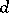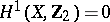# Accessibility for groups

Accessibility is concerned with bounding the complexity of decompositions as graphs of groups for a discrete group.

In his proof that groups of cohomological dimension one are necessarily free, J.R. Stallings [a13] made use of Grushko's theorem [a6], which asserts that if a groupis generated by a subset of cardinalityand decomposes as a non-trivial free product, then.

In attempting to generalize the above Stallings' theorem to pairs of relative cohomological dimension one, C.T.C. Wall [a14] conjectured that there was a similar bound on decompositions as a finitely-generated group as non-trivial amalgamated free products and HNN-extensions (cf. HNN-extension) over finite subgroups [a14]. Specifically, Wall defined a groupto be accessible if there is a positive integersuch that any reduced decomposition as a graph of groups forwith finite edge groups has at mostedges. He conjectured that all finitely-generated groups are accessible.

In a torsion-free group (cf. also Group without torsion), the only allowed decompositions are free products so it follows from Grushko's theorem that finitely-generated torsion-free groups are accessible.

Despite considerable interest in Wall's conjecture, which motivated much progress in the understanding of splittings of groups, it took until the 1990s before M.J. Dunwoody found a counterexample [a3]. In the meantime several important classes of groups were shown to be accessible. Using algebraic techniques, P.A. Linnell showed that any finitely-generated group with a bound on the order of its finite subgroups is accessible [a8], and, using geometric methods, Dunwoody proved Wall's conjecture for the class of almost finitely-presented groups, which contains as a subclass all finitely-presented groups [a4].

Much of the development of the theory of group splittings is parallel to the theory of incompressible embedded surfaces in three-dimensional manifolds (cf. also Three-dimensional manifold); for example, the analogue of Grushko's theorem is Kneser's theorem [a7] concerning embedded-incompressible-spheres in compact orientable three-dimensional manifolds (cf. also Kneser theorem).

Incompressible surfaces in three-dimensional manifolds are now (as of 2000) largely understood, using the theory of surfaces of least area [a9]. Dunwoody's proof of accessibility for finitely-presented groups uses a combinatorial analogue of this idea, which applies to group actions on a simply connected-complex, or more generally any-complexsuch that. He showed that any splitting of a groupover a finite subgroup gives rise to the existence of a minimal track in any such complex on whichacts. Reduced decompositions as a graph of groups forwith finite edge groups give rise to non-parallel disjoint tracks in the-complex via a method known as resolution. Dunwoody showed that ifacts co-compactly on the-complex, then there is a bound on the number of such disjoint tracks, and this establishes the accessibility of. Applying the method to the Cayley complex of a finitely-presented group proves accessibility in this case.

There have been generalizations of the notion of accessibility to cover decompositions over other classes of subgroups. M. Bestvina and M. Feighn were able to show that for a finitely-presented groupthere is a bound on the number of vertices in any reduced decomposition as a graph of groups forwith small edge groups [a1]. The technical notion of a "small subgroup" used here includes the case of splittings over Abelian subgroups.

Another approach to accessibility was pioneered by Z. Sela [a11]. His definition is most naturally understood using Bass–Serre theory, which gives a duality between decompositions as graphs of groups for a groupand actions ofon trees which have no global fixed point [a12].

Sela defines a decomposition as a graph of groups to be-acylindrical if it is reduced, and no element offixes a path of lengthin the corresponding Bass–Serre tree. He remarks that this notion is satisfied in many natural situations, including small decompositions of torsion-free word hyperbolic groups (cf. also Hyperbolic group). His main result is that there is a bound on the number of vertices in any reduced-acylindrical splitting of a finitely-generated, torsion-free, freely indecomposable group. The bound depends only on the group and the integer. He remarks that this notion of accessibility holds for certain groups that are not accessible in the classical sense, but that the bound is not given in terms of other algebraic invariants of the group, unlike the accessibility discussed by Dunwoody and Bestvina and Feighn.

There is now (as of 2000) a much more more powerful structure theorem for decompositions of a finitely-presented group over virtually cyclic subgroups, given by the JSJ decomposition. This powerful theorem gives a natural decomposition of any finitely-presented group which encodes all the information about splittings of the group over virtually infinite cyclic subgroups, and generalizes the Jaco–Shalen–Johansson decomposition theorem for embedded tori in three-dimensional manifolds.

It was initially proved in [a10] for the class of word hyperbolic groups, while [a2] gives a geometric derivation of the JSJ decomposition for finitely-presented groups using tracks in-complexes.

The theorem asserts that any finitely-presented groupadmits a canonical decomposition as a graph of groups in which all edge groups are virtually infinite cyclic, and any virtually cyclic subgroup over whichsplits lies in a subgroup isomorphic to a finite extension of a surface group and which is conjugate to a vertex group of the decomposition.